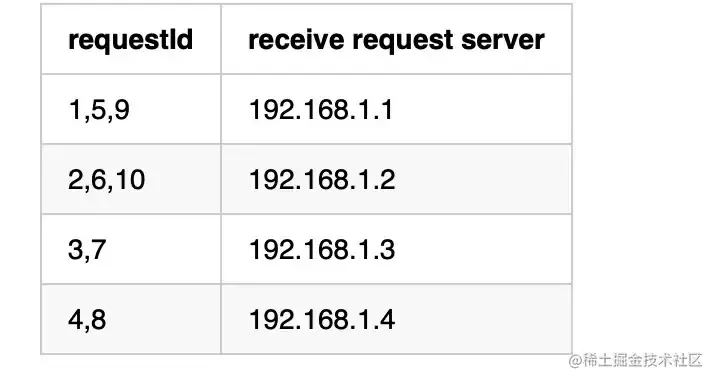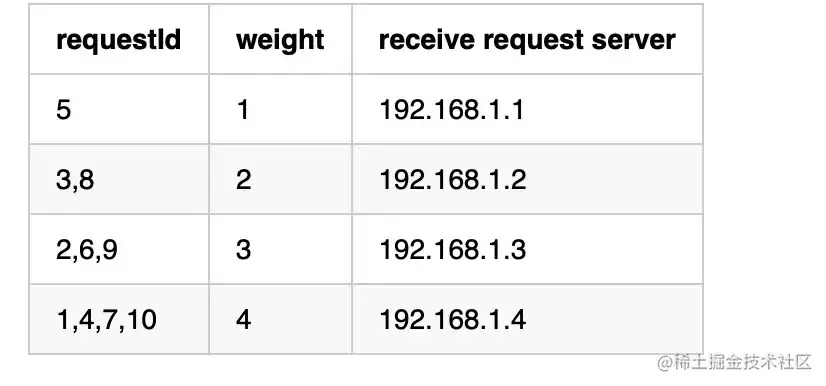# 每个Java程序员都必须知道的四种负载均衡算法_Java知识库

## 随机算法

``````public class RandomTest {

private static final List<String> servers = Arrays.asList("192.168.1.1", "192.168.1.2", "192.168.1.3", "192.168.1.4");

public static String getServer() {
Random random = new Random();
int index = random.nextInt(servers.size());
return servers.get(index);
}

public static void main(String[] args) {
for (int i = 0; i < 10; i++) {
String server = getServer();
System.out.println("select server: "+server);
}
}
}

## 轮询算法

`Round-Robin`轮询算法是另一种经典的负载均衡算法。请求以循环的方式分发到集群中的所有服务器。同理，对于上述四台服务器，假设客户端向集群发送10个请求，则请求分布将如下图所示：## 加权轮询算法

`Weighted Round-Robin`加权轮询算法是在`round-robin`算法的基础上根据服务器的性能分配权重。服务器能支持的请求越多，权重就越高，分配的请求也就越多。对于同样的10个请求，使用加权轮询算法的请求分布会如下图所示：``````public class RoundRobinTest {

public class Node{
private String ip;

private Integer weight;

private Integer currentWeight;

public Node(String ip,Integer weight) {
this.ip = ip;
this.weight = weight;
this.currentWeight = weight;
}

public String getIp() {
return ip;
}

public void setIp(String ip) {
this.ip = ip;
}

public Integer getWeight() {
return weight;
}

public void setWeight(Integer weight) {
this.weight = weight;
}

public Integer getCurrentWeight() {
return currentWeight;
}

public void setCurrentWeight(Integer currentWeight) {
this.currentWeight = currentWeight;
}
}

List<Node> servers = Arrays.asList(
new Node("192.168.1.1",1),
new Node("192.168.1.2",2),
new Node("192.168.1.3",3),
new Node("192.168.1.4",4));
private Integer totalWeight;

public RoundRobinTest() {
this.totalWeight = servers.stream()
.mapToInt(Node::getWeight)
.reduce((a,b)->a+b).getAsInt();
}

public String getServer() {
Node node = servers.stream().max(Comparator.comparingInt(Node::getCurrentWeight)).get();
node.setCurrentWeight(node.getCurrentWeight()-totalWeight);
servers.forEach(server->server.setCurrentWeight(server.getCurrentWeight()+server.getWeight()));
return node.getIp();
}

public static void main(String[] args) {
RoundRobinTest roundRobinTest = new RoundRobinTest();
for (int i = 0; i < 10; i++) {
String server = roundRobinTest.getServer();
System.out.println("select server: "+server);
}
}

## 哈希算法

``````rivate static final List<String> servers = Arrays.asList("192.168.1.1", "192.168.1.2", "192.168.1.3", "192.168.1.4");

public static String getServer(String key) {
int hash = key.hashCode();
int index =  hash%servers.size();
return servers.get(index);
}

public static void main(String[] args) {
for (int i = 0; i < 10; i++) {
String server = getServer(String.valueOf(i));
System.out.println("select server: "+server);
}
}